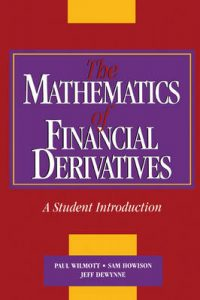##### June 5, 2023# The Mathematics of Financial Derivatives

## The Mathematics of Financial DerivativesFinance is one of the fastest growing areas in the modern banking and corporate world. This, together with the sophistication of modern financial products, provides a rapidly growing impetus for new mathematical models and modern mathematical methods; the area is an expanding source for novel and relevant ‘real-world’ mathematics. In this book the authors describe the modelling of financial derivative products from an applied mathematician’s viewpoint, from modelling through analysis to elementary computation. A unified approach to modelling derivative products as partial differential equations is presented, using numerical solutions where appropriate. Some mathematics is assumed, but clear explanations are provided for material beyond elementary calculus, probability, and algebra. Over 140 exercises are included. This volume will become the standard introduction to this exciting new field for advanced undergraduate students.